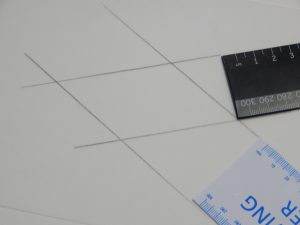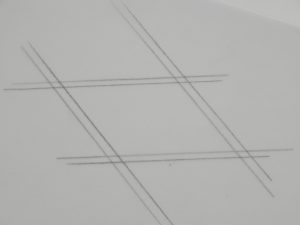We start from this definition:

A parallelogram is a quadrilateral with both pairs of opposite edges parallel.

In this investigation you will discover some other properties of parallelograms.

Step 1: Use two different width rulers to draw a parallelogram on tracing or baking paper.Make sure that it is not a rhombus and that the adjacent edges are not equal in length.

Step 2: Place a second piece of tracing paper over the first and copy the parallelogram.

Step 3: Slide the second tracing paper over the first to compare the lengths of the opposite edges of the parallelogram.

How do the lengths of the opposite edges compare?

What did you discover? Call this Conjecture 1: Both pairs of opposite edges of a parallelogram are ….

Step 4: Rotate or flip the second paper to compare the sizes of the opposite angles of the parallelogram.

How do the sizes of the opposite angles compare?

What did you discover?  Call this Conjecture 2: Both pairs of opposite angles of a parallelogram are …

Step 5: What can you discover about the point of intersection of the diagonals? Draw or fold the two diagonals of the parallelogram. Place a dot on their intersection.

Fold to compare the lengths of the two segments on each diagonal.

What did you discover? Call this Conjecture 3. It could be: The point of intersection of the diagonals of a parallelogram …

Step 6: Now fold the diagonals of one of the sheets and compare angles between the diagonals. Are the angles equal in size?